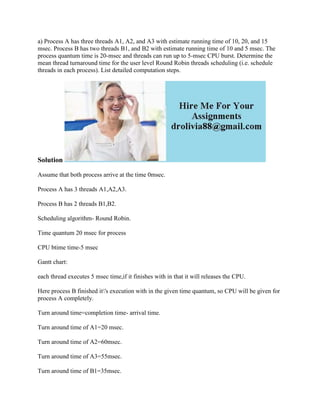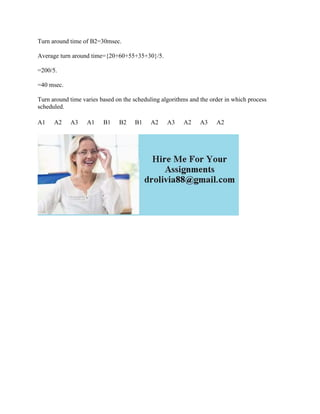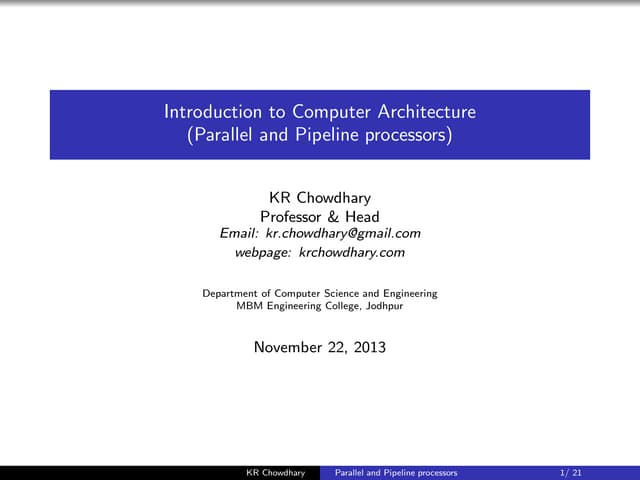Anúncio

# a) Process A has three threads A1- A2- and A3 with estimate running ti.docx

3 de Feb de 2023Próximos SlideSharespipelining ppt.pdf
Carregando em ... 3
1 de 2
Anúncio

### a) Process A has three threads A1- A2- and A3 with estimate running ti.docx

1. a) Process A has three threads A1, A2, and A3 with estimate running time of 10, 20, and 15 msec. Process B has two threads B1, and B2 with estimate running time of 10 and 5 msec. The process quantum time is 20-msec and threads can run up to 5-msec CPU burst. Determine the mean thread turnaround time for the user level Round Robin threads scheduling (i.e. schedule threads in each process). List detailed computation steps. Solution Assume that both process arrive at the time 0msec. Process A has 3 threads A1,A2,A3. Process B has 2 threads B1,B2. Scheduling algorithm- Round Robin. Time quantum 20 msec for process CPU btime time-5 msec Gantt chart: each thread executes 5 msec time,if it finishes with in that it will releases the CPU. Here process B finished it's execution with in the given time quantum, so CPU will be given for process A completely. Turn around time=completion time- arrival time. Turn around time of A1=20 msec. Turn around time of A2=60msec. Turn around time of A3=55msec. Turn around time of B1=35msec.
2. Turn around time of B2=30msec. Average turn around time={20+60+55+35+30}/5. =200/5. =40 msec. Turn around time varies based on the scheduling algorithms and the order in which process scheduled. A1 A2 A3 A1 B1 B2 B1 A2 A3 A2 A3 A2
Anúncio